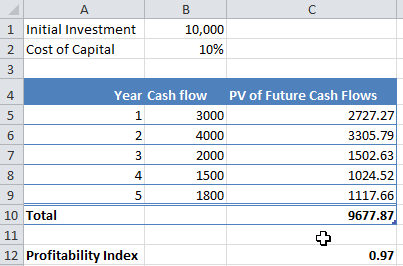# Calculating Profitability Index in Excel

Profitability index is an important measure in project finance to decide whether to invest in a project or not. It is calculated as the ratio of present value of a project cash flows and the initial investment.

$Profitability\ Index = \frac{PV\ of\ Future\ Cash\ Flows}{Initial\ Investment}$

If the profitability index is greater than 1, the project is accepted, and if it is less than 1, the project is rejected.

Let’s see how profitability index can be calculated in excel. Let us say that we are examining a project, which requires an initial investment of $10,000, and after the will give us cash flow of$3,000, $4,000,$2,000, 41,500, and \$1,800 in the next five years.

To calculate the profitability index:

Step 1: Assume a required rate of return, or cost of capital for the project. Let’s say the cost of capital is 10%.

Step 2: Calculate the present value of all future cash flows. You can use the PV() function in excel for this calculation.

Step 3: Take the total of PV of all future cash flows. In our example, the total is 9677.87.

Step 4: Calculate profitability Index as follows:

Profitability Index = 9677.87/10,000 = 0.97

Since the profitability index is less than one, this project should not be accepted. The cash flows and calculations are shown below:Member-only